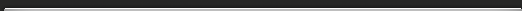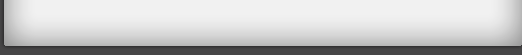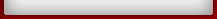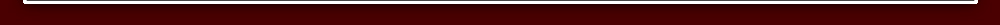Tutorial 5

## Tutorial 5

An elegant way of multiplying numbers using a simple pattern
21 x 23 = 483
This is normally called long multiplication butactually the answer can be written straight downusing the VERTICALLY AND CROSSWISE formula.
We first put, or imagine, 23 below 21:There are 3 steps:

a) Multiply vertically on the left: 2 x 2 = 4.
This gives the first figure of the answer.
b) Multiply crosswise and add: 2 x 3 + 1 x 2 = 8
This gives the middle figure.
c) Multiply vertically on the right: 1 x 3 = 3
This gives the last figure of the answer.
And thats all there is to it.
Similarly 61 x 31 = 18916 x 3 = 18; 6 x 1 + 1 x 3 = 9; 1 x 1 = 1
Exercise 1 Tutorial 5

1) 14 x 21
2) 22 x 31
3) 21 x 31
4) 21 x 22
5) 32 x 21
Exercise 2a Tutorial 5

Multiply any 2-figure numbers together by mere mental arithmetic!
If you want 21 stamps at 26 pence each you can
There were no carries in the method given above.,
However, there only involve one small extra step.
21 x 26 = 546The method is the same as above except that we get a 2-figure number, 14, in the middle step, so the 1 is carried over to the left (4 becomes 5).
So 21 stamps cost 5.46.
Practise a few:
1) 21 x 47
2) 23 x 43
3) 32 x 53
4) 42 x 32
5) 71 x 72
Exercise 2b Tutorial 5

33 x 44 = 1452
There may be more than one carry in a sum:Vertically on the left we get 12.
Crosswise gives us 24, so we carry 2 to the left
and mentally get 144.
Then vertically on the right we get 12 and the 1
here is carried over to the 144 to make 1452.

6) 32 x 56
7) 32 x 54
8) 31 x 72
9) 44 x 53
10) 54 x 64

Any two numbers, no matter how big, can be multiplied in one line by this method.